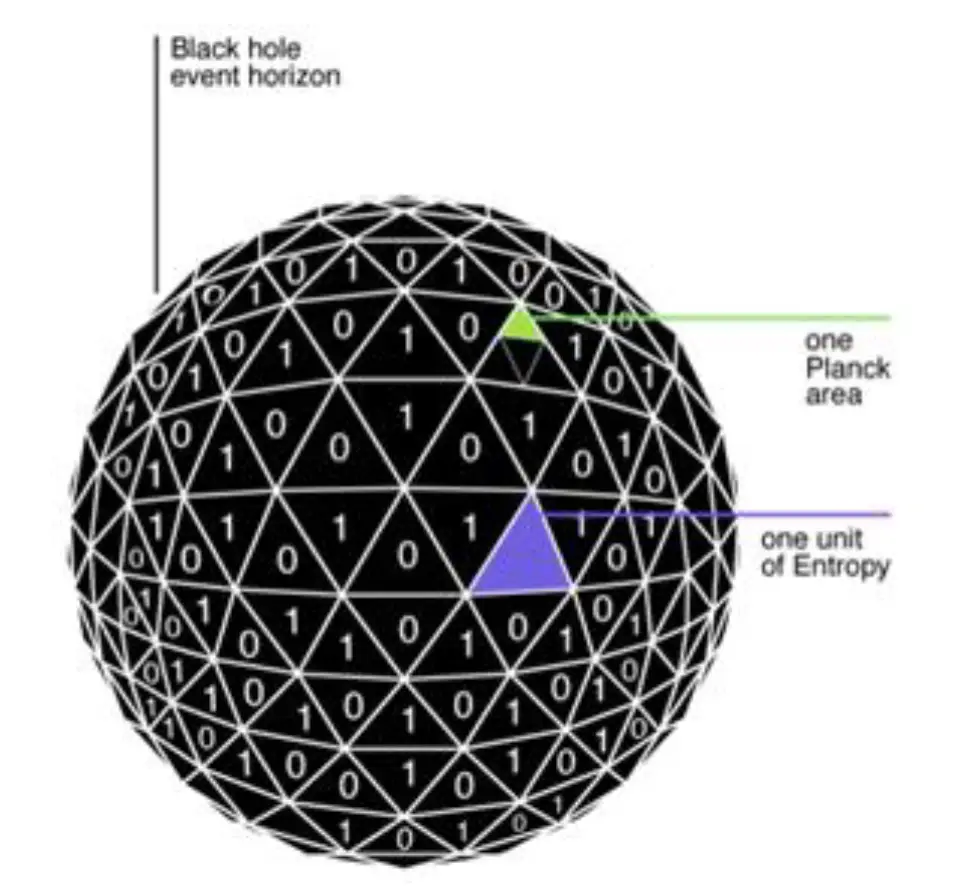# Black Hole Physics

In physics, an upper limit on the thermodynamic entropy S, or Shannon entropy H, that can be contained within a given finite region of space which has a finite amount of energy is the Bekenstein boundor conversely, the maximal amount of information required to perfectly describe a given physical system down to the quantum level.

Furthermore, generally, the entropy is proportional to the number of bits necessary to describe the state of the system considered.

This result, which was demonstrated by Jacob Bekenstein corresponds to the interpretation in terms of bits of information.

$$S_{BH}$$=$$k \over 4$$($$c^3 \over ħG$$)A=k$$A \over l^2_p$$=S=k ln(W)

SBH= Bekenstein-Hawking  entropy (black hole), KB=Boltzmann constant, c=speed of light,  ħ= Reduced Planck's constant, G=Gravitational Constant, A=area of the event horizon of the black hole, L²p= Planck surface, S= entropy Boltzmann,  W = microstates.

According to the Bekenstein bound, the entropy of a black hole is proportional to the number of Planck areas that it would take to cover the black hole’s event horizonThe Planck surface intervenes in particular in the thermodynamics of black holes, where it corresponds to a quantity of information

The Planck surface is a specific expression for black-hole entropy in terms of black-hole area.

The Planck surface is the quarter of the area by which the horizon of a spherical black hole increases when it absorbs a bit of information

We take the area of a black hole as a measure of its entropy entropy in the sense of inaccessibility of information about its internal configuration

The thermodynamics of black holes suggests certain relationships between the entropy of black holes and their geometry.

The universal form of the bound was originally found by Jacob Bekenstein in 1981 as the inequality

$$S<$$$$2πkRE \over ħc$$

The Bekenstein bound corresponds to the interpretation in terms of bits of information of a given physical system down to the quantum level.

where S is the entropy, k is Boltzmann’s constant, R is the radius of a sphere that can enclose the given system, E is the total massenergy including any rest masses, ħ is the reduced Planck constant, and c is the speed of light.

Note that while gravity plays a significant role in its enforcement, the expression for the bound does not contain the gravitational constant G.

In
informational terms, the relation between thermodynamic entropy S and Shannon entropy H is given by relation between S & H

$$S=$$$$kHln(2)$$

$$H=<$$$$2πRE \over ħcln(2)$$

where H is the Shannon entropy expressed in number of bits contained in the quantum states in the sphere.

The ln 2 factor comes from defining the information as the logarithm to the base 2 of the number of quantum states.

The choice of logarithmsstatistical functions almost always use logarithms to scale down ratios and large numbers towards a common range representable between 0 and 1.

It is then natural to introduce the concept of black-hole entropy as the measure of the inaccessibility of information (to an exterior observer) as to which particular internal configuration of the black hole.